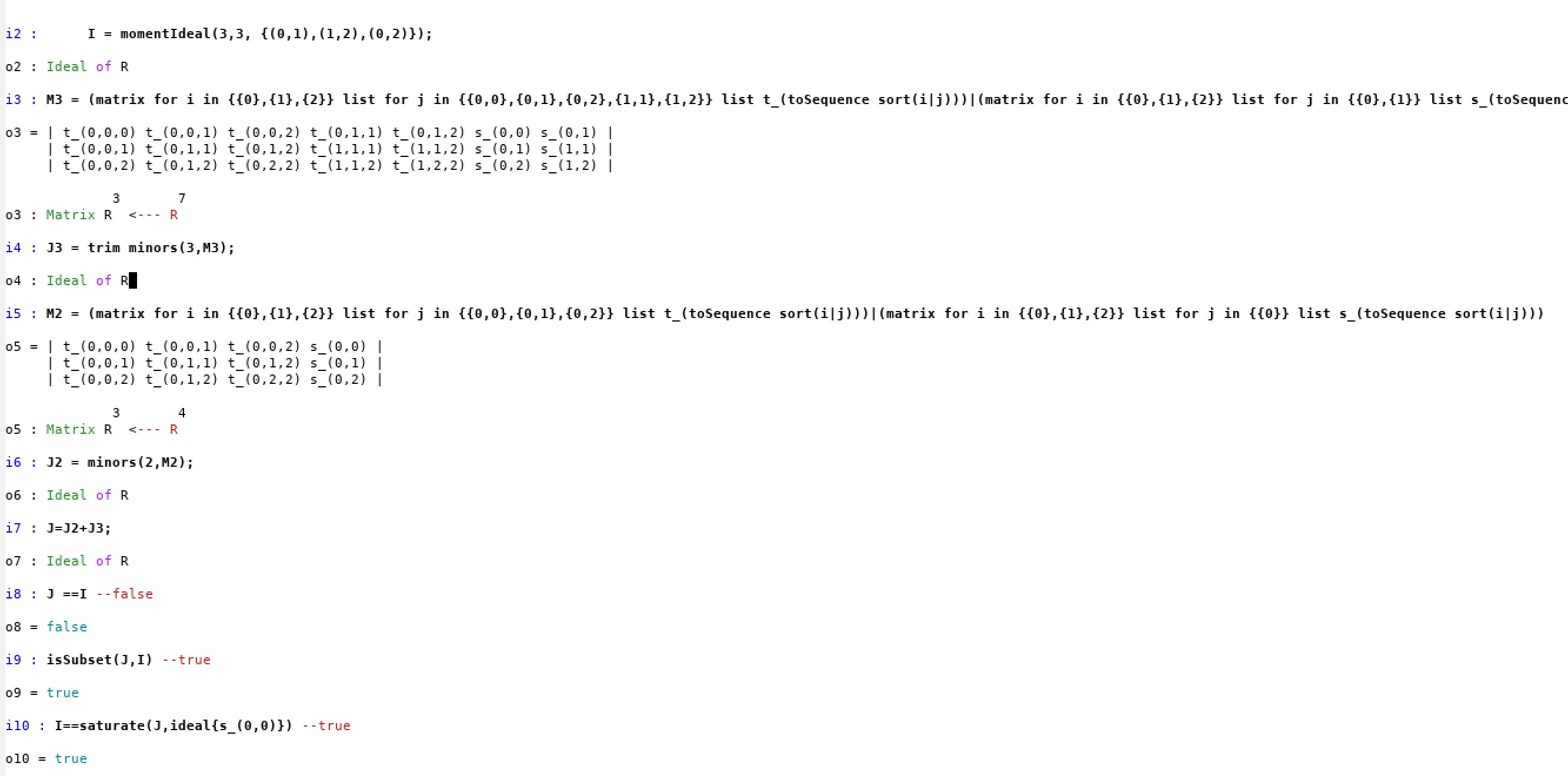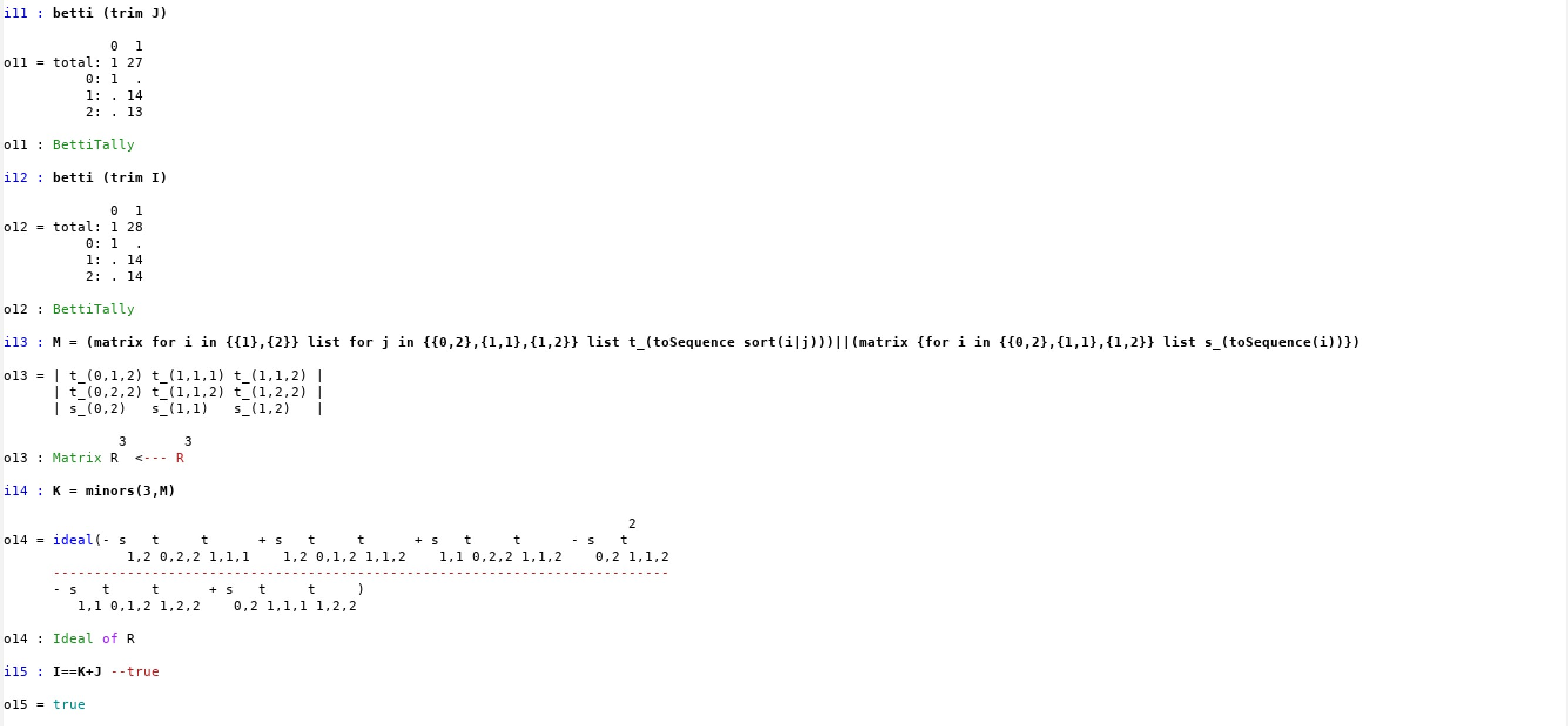# The unexplored forest of non-trees

In this site we reproduce the computations in Macaulay2 regarding all non-tree examples of our paper (Section 7).

The full code can be found in Non-trees.

The function below computes the vanishing ideal of the model $$\mathcal{M}^{\leq 3}(G)$$ via the trek parametrization (as opposed to the simple trek parametrization described in Section 2). In this way, we can compute the ideal even if $$G$$ has a cyclic structure.

-- n=number of vertices
-- k=higher moment that we want to compute
-- edges: list of edges
-- WARNING: numbering of vertices starts with 0

momentIdeal = (n, k, edges) -> (
list2 = flatten for i to n-1 list for j from i to n-1 list (i,j);
list3 = flatten flatten for i to n-1 list for j from i to n-1 list for k from j to n-1 list (i,j,k);
R = QQ[l_(0,0)..l_(n-1,n-1), apply(list2,i->s_i),apply(list3,i->t_i)];
L = mutableIdentity(R, n);
for e in edges do (
L_e = -l_e;
);
L = matrix(L);
L =  substitute(L, R);
use R;
S = matrix( apply(n, i -> apply(n, j -> if (i < j) then s_(i,j) else s_(j,i))));
W = (transpose L)*S*L;
equationList = flatten for i to n-1 list for j from i+1 to n-1 list W_(i,j);
for i from 0 to n-1 do (
for j from 0 to n-1 do (
for k from 0 to n-1 do (
use R;
if(i == j and i == k) then continue;
eqn = sum(apply(n, a -> sum(apply(n, b -> sum(apply(n, c -> L_(a,i)*L_(b,j)*L_(c,k)*t_(toSequence sort{a,b,c})))))));
equationList = append(equationList, eqn);
);
);
);
I = ideal(equationList);
Isat = saturate(I, ideal(det(L)));
J = eliminate(toList(l_(0,0)..l_(n-1,n-1)), Isat);
return(J);
);


Example 7.1 Let $$G$$ be DAG with edges $$0\rightarrow 1,\,1\rightarrow 2,\,0\rightarrow 2$$. We compute the vanishing ideal after running the previous function and compare $$\mathcal{I}^{\leq 3}(G)$$ with the ideal of special 2 and 3-minors arising from trek-matrices.

I = momentIdeal(3,3, {(0,1),(1,2),(0,2)});

M3 = (matrix for i in {{0},{1},{2}} list for j in {{0,0},{0,1},{0,2},{1,1},{1,2}} list t_(toSequence sort(i|j)))|(matrix for i in {{0},{1},{2}} list for j in {{0},{1}} list s_(toSequence sort(i|j)))
J3 = trim minors(3,M3);

M2 = (matrix for i in {{0},{1},{2}} list for j in {{0,0},{0,1},{0,2}} list t_(toSequence sort(i|j)))|(matrix for i in {{0},{1},{2}} list for j in {{0}} list s_(toSequence sort(i|j)))
J2 = minors(2,M2);
J=J2+J3;
J ==I --false
isSubset(J,I) --true
I==saturate(J,ideal{s_(0,0)}) --true
-- J is strictly contained in I but equal up to saturation w.r.t. s_(0,0)Next we obtain all generators of the ideal by allowing the emptyset in the row labeling.

betti (trim J)
betti (trim I)

-- There's a single cubic polynomial that does not arise as minor of the previous matrices:

M = (matrix for i in {{1},{2}} list for j in {{0,2},{1,1},{1,2}} list t_(toSequence sort(i|j)))||(matrix {for i in {{0,2},{1,1},{1,2}} list s_(toSequence(i))})
K = minors(3,M)

I==K+J --trueExample 7.3 Let $$G$$ be the 2-cycle, namely the directed graph with edges $$1\rightarrow 2,\,2\rightarrow 1$$. We can compute the vanishing ideal by inputing the following code:

I = momentIdeal(2,3, {(1,2),(2,1)})
dim I
degree I


Example 7.4 Let $$G$$ be the 3-cycle. In this case the computations are very expensive and it had to run on a server (technical details: Intel Xeon(R) CPU E5-2680 v4 2.40GHz × 56 processors, run with 300 Gb memory).

I = momentIdeal(2,3, {(1,2),(2,3),(3,1)})
dim I
degree I